UIHistories Project: A History of the University of Illinois by Kalev Leetaru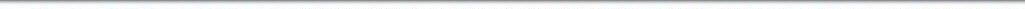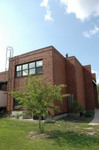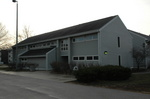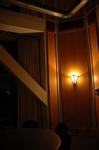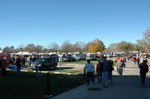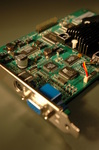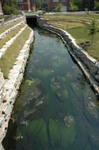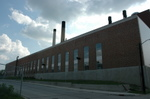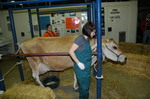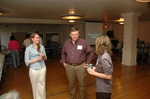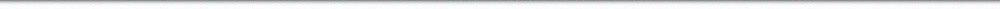N A V I G A T I O N D I G I T A L L I B R A R Y

## Repository: UIHistories Project: Board of Trustees Minutes - 1896 [PAGE 86]

Caption: Board of Trustees Minutes - 1896
This is a reduced-resolution page image for fast online browsing.

< Previous Page [Displaying Page 86 of 371] Next Page >
[VIEW ALL PAGE THUMBNAILS]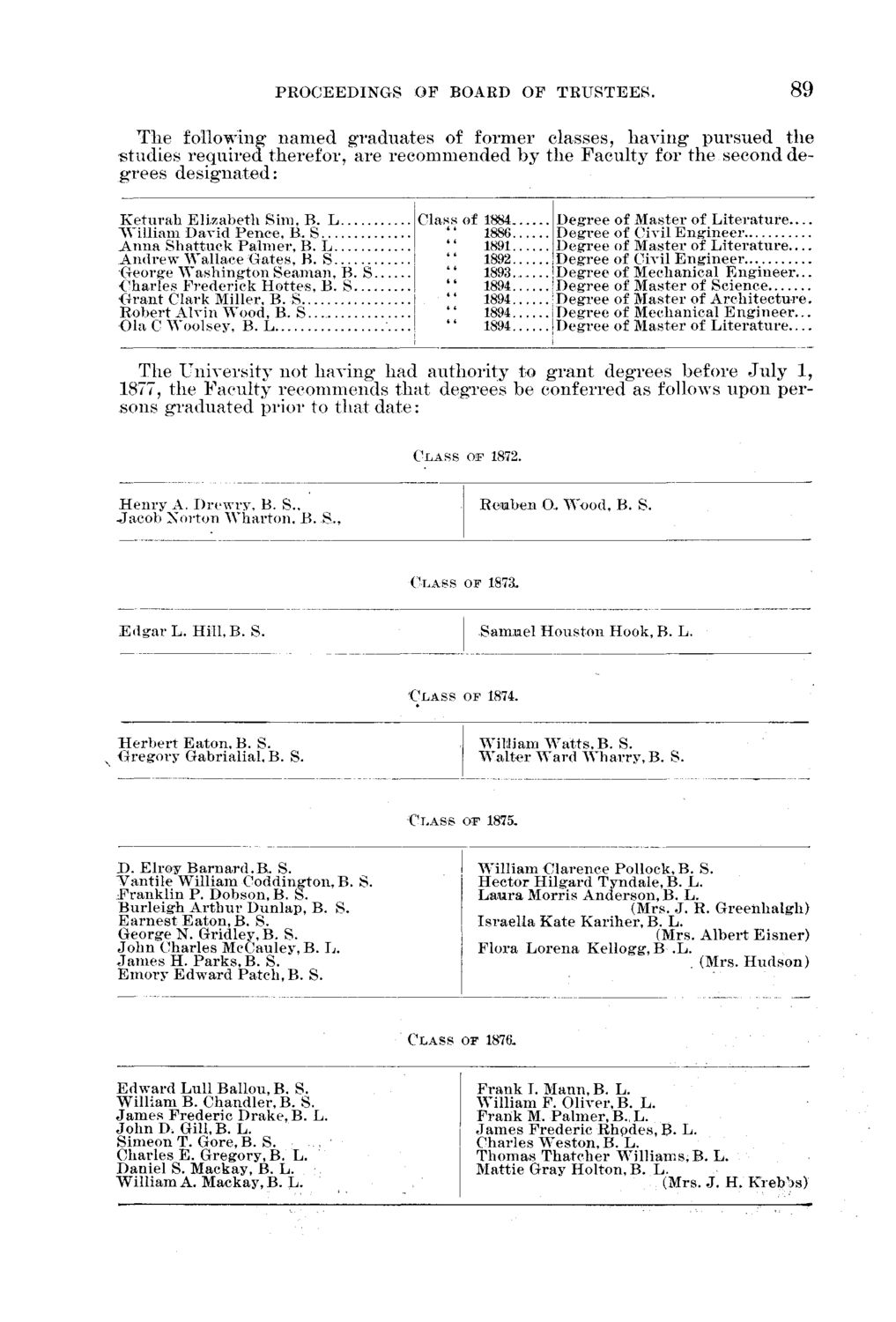### EXTRACTED TEXT FROM PAGE:

PROCEEDINGS OF BOARD OF TRUSTEES.

89

The following named graduates of former classes, having* pursued the studies required therefor, are recommended by the Faculty for the second degrees designated:

K e t u r a h Elizabeth Sim, B. L "William David Pence, B. S A n n a Shattuck Palmer, B. L A n d r e w Wallace Gates, B. S George W a s h i n g t o n Seaman, B. S •Charles Frederick Hottes, B . S G r a n t Clark Miller, B. S Robert Alvin Wood, B, S Ola C Woolsey, B. L Class of 1884 1886 1891 1892 1893 1894 1894 1894 1894 Degree Degree Degree Degree Degree Degree Degree Degree Degree of of of of of of of of of Master of L i t e r a t u r e — Civil E n g i n e e r Master of L i t e r a t u r e — Civil E n g i n e e r Mechanical E n g i n e e r . . . Master of Science Master of A r c h i t e c t u r e . Mechanical E n g i n e e r . . . Master of L i t e r a t u r e . . . .

The University not having had authority to grant degrees before July 1, 1877, the Faculty recommends that degrees be conferred as follows upon persons graduated prior to that date:

C L A S S oap 1872.

H e n r y A. Drewry, B. S., J a c o b Norton W h a r t o n . B .

Reuben O. Wood, B. S.

C L A S S OF

187a.

E d g a r L. H i l l , B . S.

Samuel Houston Hook, B. L.

C L A S S OF

1874.

H e r b e r t Eaton, B. S. Gregory Gabrialial, B. S.

William W a t t s , B. S. W a l t e r W a r d W h a r r y , B. S.

C L A S S OIF 1875.

D . Elroy B a r n a r d . B . S. P a n t i l e William Coddington,B. S. F r a n k l i n P. Dobson, B. S. Burleigrh A r t h u r Dunlap, B. S. E a r n e s t Eaton, B. S. George N . G r i d l e y , B . S. J o h n Charles McCauley, B. L. J a m e s H. P a r k s , B. S. E m o r y E d w a r d Patch, B. S.

William Clarence Pollock, B. S. Hector Hilgard Tyndale, B. L. L a u r a Morris Anderson, B. L. (Mrs. J. R. Greeiihalgh) Israella Kate Kariher, B. L. (Mrs. Albert E i s n e r ) Flora Lorena Kellog-g, B .L. (Mrs. H u d s o n )

C L A S S OF

1876.

E d w a r d Lull Ballou, B. S. William B. Chandler, B. S. J a m e s Frederic Drake, B. L. J o h n D . Gill,B. L. Simeon T. Gore, B. S. , Charles E. Gregory, B. L. Daniel S. Mackay, B. L. William A. Mackay, B. L.

F r a n k I. Mann, B. L. William F. Oliver, B. L. F r a n k M. Palmer, B., L. J a m e s Frederic Rhpdes, B. L. Charles Weston, B. L. Thomas T h a t c h e r W i l l i a m s , B . L. Mattie Gray Holton, B. L. (Mrs. J. H. Krebbs)